# ode113

## 语法

[t,y] = ode113(odefun,tspan,y0)
[t,y] = ode113(odefun,tspan,y0,options)
[t,y,te,ye,ie] = ode113(odefun,tspan,y0,options)
sol = ode113(___)

## 说明

[t,y] = ode113(odefun,tspan,y0)（其中 tspan = [t0 tf]）求微分方程组 $y\text{'}=f\left(t,y\right)$ 从 t0 到 tf 的积分，初始条件为 y0。解数组 y 中的每一行都与列向量 t 中返回的值相对应。所有 MATLAB® ODE 求解器都可以解算 $y\text{'}=f\left(t,y\right)$ 形式的方程组，或涉及质量矩阵 $M\left(t,y\right)y\text{'}=f\left(t,y\right)$ 的问题。求解器都使用类似的语法。ode23s 求解器只能解算质量矩阵为常量的问题。ode15s 和 ode23t 可以解算具有奇异质量矩阵的问题，称为微分代数方程 (DAE)。使用 odeset 的 Mass 选项指定质量矩阵。

[t,y] = ode113(odefun,tspan,y0,options) 还使用由 options（使用 odeset 函数创建的参数）定义的积分设置。例如，使用 AbsTol 和 RelTol 选项指定绝对误差容限和相对误差容限，或者使用 Mass 选项提供质量矩阵。
[t,y,te,ye,ie] = ode113(odefun,tspan,y0,options) 还求 (t,y) 的函数（称为事件函数）在何处为零。在输出中，te 是事件的时间，ye 是事件发生时的解，ie 是触发的事件的索引。对于每个事件函数，应指定积分是否在零点处终止以及过零方向是否重要。为此，请将 'Events' 属性设置为函数（例如 myEventFcn 或 @myEventFcn），并创建一个对应的函数：[value,isterminal,direction] = myEventFcn(t,y)。有关详细信息，请参阅 ODE 事件位置。
sol = ode113(___) 返回一个结构体，您可以将该结构体与 deval 结合使用来计算区间 [t0 tf] 中任意点位置的解。您可以使用上述语法中的任何输入参数组合。

## 示例

${y}^{\prime }=2t.$

tspan = [0 5]; y0 = 0; [t,y] = ode113(@(t,y) 2*t, tspan, y0);

plot(t,y,'-o')van der Pol 方程为二阶 ODE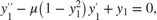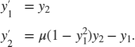function dydt = vdp1(t,y) %VDP1 Evaluate the van der Pol ODEs for mu = 1 % % See also ODE113, ODE23, ODE45. % Jacek Kierzenka and Lawrence F. Shampine % Copyright 1984-2014 The MathWorks, Inc. dydt = [y(2); (1-y(1)^2)*y(2)-y(1)]; 

[t,y] = ode113(@vdp1,[0 20],[2; 0]); 

plot(t,y(:,1),'-o',t,y(:,2),'-o') title('Solution of van der Pol Equation (\mu = 1) with ODE113'); xlabel('Time t'); ylabel('Solution y'); legend('y_1','y_2')ode113 仅适用于使用两个输入参数（ty）的函数。但是，通过在函数外部定义参数并在指定函数句柄时传递这些参数，可以传入额外参数。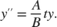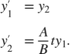odefcn.m 将此方程组表示为接受四个输入参数（tyAB）的函数。

function dydt = odefcn(t,y,A,B) dydt = zeros(2,1); dydt(1) = y(2); dydt(2) = (A/B)*t.*y(1); 

A = 1; B = 2; tspan = [0 5]; y0 = [0 0.01]; [t,y] = ode113(@(t,y) odefcn(t,y,A,B), tspan, y0); 

plot(t,y(:,1),'-o',t,y(:,2),'-.')ode45 相比，ode113ode78ode89 求解器可以更好地求解具有严格误差容限的问题。ode113 更胜一筹的常见情况是在轨道动力学问题中，这些问题的解曲线是平滑的且需要很高的准确性。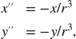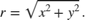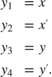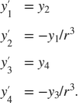function dy = twobodyode(t,y) % Two body problem with one mass much larger than the other. r = sqrt(y(1)^2 + y(3)^2); dy = [y(2); -y(1)/r^3; y(4); -y(3)/r^3]; 

opts = odeset('Reltol',1e-13,'AbsTol',1e-14,'Stats','on'); tspan = [0 10*pi]; y0 = [2 0 0 0.5]; [t,y] = ode113(@twobodyode, tspan, y0, opts); plot(t,y) legend('x','x''','y','y''','Location','SouthEast') title('Position and Velocity Components') 
924 successful steps 4 failed attempts 1853 function evaluationsfigure plot(y(:,1),y(:,3),'-o',0,0,'ro') axis equal title('Orbit of Smaller Mass')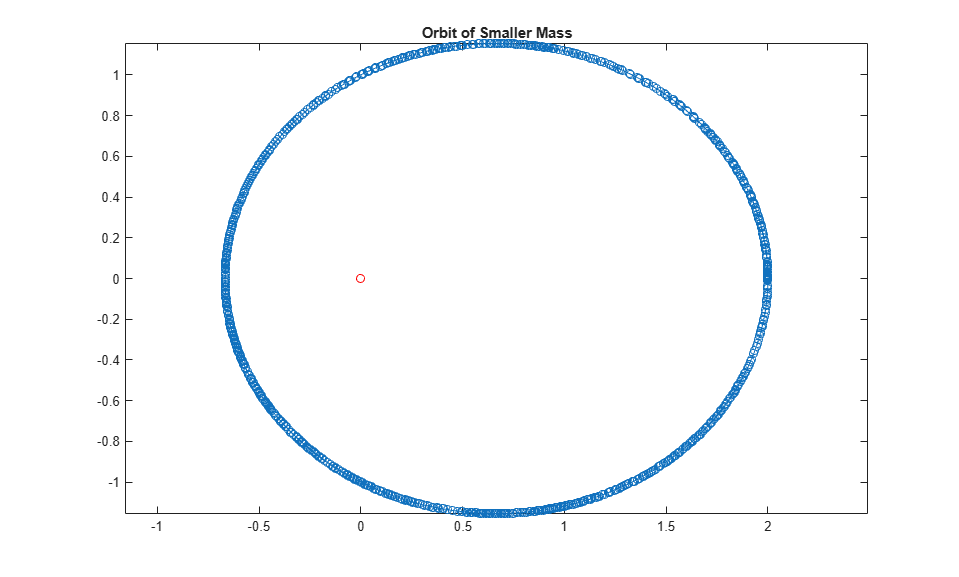ode45 相比，ode113 求解器能够通过更少的函数计算更快地获得解。

## 输入参数

function dydt = odefun(t,y) dydt = 5*y-3; end

$\begin{array}{l}y{\text{'}}_{1}={y}_{1}+2{y}_{2}\\ y{\text{'}}_{2}=3{y}_{1}+2{y}_{2}\end{array}$

function dydt = odefun(t,y) dydt = zeros(2,1); dydt(1) = y(1)+2*y(2); dydt(2) = 3*y(1)+2*y(2); end

• 如果 tspan 有两个元素 [t0 tf]，求解器将返回在该区间内的每个内部积分步计算的解。

• 如果 tspan 包含两个以上的元素，[t0,t1,t2,...,tf]，求解器将返回在给定点处计算的解。但是，求解器不会精确步进到 tspan 中指定的每个点。此时，求解器使用自己的内部积分步来计算解，然后在 tspan 中请求的各点处计算解。在指定点处生成的解与在每个内部积分步计算的解具有相同的准确度级别。

指定多个中间点对计算效率影响甚微，但对于大型系统可能会影响内存管理。

• 如果 tspan 包含多个中间点 [t0,t1,t2,...,tf]，则指定的点表示了问题的规模，这可能影响求解器使用的 InitialStep 的值。因此，根据您是将 tspan 指定为二元素向量还是包含中间点的向量，求解器获得的解可能有所不同。

• tspan 中的初始值和最终值用于计算最大步长 MaxStep。因此，更改 tspan 中的初始值或最终值可能导致求解器使用不同步长序列，从而可能会更改解。

options 结构体，指定为结构体数组。使用 odeset 函数创建或修改 options 结构体。有关与每个求解器兼容的选项列表，请参阅 ODE 选项摘要

## 输出参数

• 如果 tspan 包含两个元素 [t0 tf]，则 t 包含用于执行积分的内部计算点。

• 如果 tspan 包含两个以上元素，则 ttspan 相同。

sol.x

sol.y

sol.solver

sol.xe

sol.ye

sol.xe 中的事件相对应的解。

sol.ie

Events 选项中指定的函数所返回的向量的索引。这些值指示求解器检测到的事件。

## 算法

ode113 是一个可变步长、可变阶次 (VSVO) 的 Adams-Bashforth-Moulton PECE 求解器，阶次从 1 到 13。使用的最高阶次似乎是 12，但 13 阶公式用于形成误差估计，并且该函数会进行局部外插，以便继续完成第 13 阶的积分。

 Shampine, L. F. and M. K. Gordon, Computer Solution of Ordinary Differential Equations: the Initial Value Problem, W. H. Freeman, San Francisco, 1975.

 Shampine, L. F. and M. W. Reichelt, “The MATLAB ODE Suite,” SIAM Journal on Scientific Computing, Vol. 18, 1997, pp. 1–22.